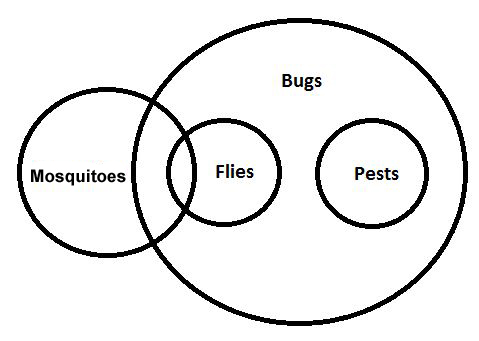Statement & Conclusion Questions for RRB Group-D PDF

0
1039

Statement & Conclusion Questions for RRB Group-D PDF

Download Top-15 RRB Group-D Statement & Conclusion Questions PDF. RRB GROUP-D Statement & Conclusion questions based on asked questions in previous exam papers very important for the Railway Group-D exam.

Question 1: In the following question, some statements are given followed by two conclusions. Decide which of the given conclusions logically follows from the given statements and then pick the answer accordingly.
Statement 1: All apples are mangoes
Statement 2: Some mangoes are oranges
Statement 3: Some oranges are fruits
Conclusion 1: Some apples are fruits
Conclusion 2: Some apples are not fruits

a) Only conclusion 1 follows

b) Only conclusion 2 follows

c) Both conclusions follow

d) Either conclusion 1 or conclusion 2 follows

Question 2: In the following question, some statements are given followed by two conclusions. Decide which of the given conclusions logically follows from the given statements and then pick the answer accordingly.
Statement 1: Some books are papers
Statement 2: All papers are pens
Conclusion 1: Some books are pens
Conclusion 2: No paper is a book

a) Only conclusion 1 follows

b) Only conclusion 2 follows

c) Both conclusions follow

d) Neither conclusion follows

Question 3: In the question below are statements followed by two conclusions numbered I and II. You have to take the two given statements to be true even if they seem to be at variance from commonly known facts and then decide which of the given conclusions logically follows from the given statements disregarding commonly known facts?
Some barks are trees.
Some trees are plants.
Conclusion:
I) Some barks are plants.
II) All trees are plants.

a) If only Conclusion I follows

b) If only Conclusion II follows

c) If neither Conclusion I nor II follows

d) If both Conclusions I and II follow

Question 4: In the question below are statements followed by two conclusions numbered I and II. You have to take the two given statements to be true even if they seem to be at variance from commonly known facts and then decide which of the given conclusions logically follows from the given statements disregarding commonly known facts?
All bats are wickets.
All balls are bats.
Conclusion:
I) All balls are wickets.
II) All wickets are bats.

a) If only Conclusion I follows

b) If only Conclusion II follows

c) If neither Conclusion I nor II follows

d) If both Conclusions I and II follow

Question 5: Statement: In a football match between the teams A and B, team A scored 8 goals, out of which 6 goals were scored by mid-fielders.
Conclusion:
I) Mid-fielders are the nearest people to the goal post.
II) 75% of team A are mid-fielders

a) Only conclusion I follows

b) Only conclusion II follows

c) Neither I nor II follows

d) Both I and II follow

Question 6: In the question below are statements followed by two conclusions numbered I and II. You have to take the two given statements to be true even if they seem to be at variance from commonly known facts and then decide which of the given conclusions logically follows from the given statements disregarding commonly known facts?
Statements:All apples are fruits. All fruits are flowers.
Conclusions:1)All apples are flowers
2)Some apples are not flowers

a) Only Conclusion 1 follows.

b) Only Conclusion 2 follows.

c) Both Conclusion 1 and Conclusion 2 follows.

d) Neither Conclusion 1 nor Conclusion 2 follows.

Question 7: In the question below are statements followed by two conclusions numbered I and II. You have to take the two given statements to be true even if they seem to be at variance from commonly known facts and then decide which of the given conclusions logically follows from the given statements disregarding commonly known facts?
Statements:
Some actors are creative.
Some Creative are genius.
Conclusions:
1) Some genius are actors.
2) No genius is an actor

a) Only Conclusion 1 follows.

b) Only Conclusion 2 follows.

c) Both Conclusion 1 and Conclusion 2 follows.

d) Either Conclusion 1 or Conclusion 2 follows.

Question 8: In the question below are statements followed by two conclusions numbered I and II. You have to take the two given statements to be true even if they seem to be at variance from commonly known facts and then decide which of the given conclusions logically follows from the given statements disregarding commonly known facts?
Statements:All apples are oranges. No mango is an orange.
Conclusions:1)Some apples are mangoes.2)All apples are mangoes.

a) Only Conclusion 1 follows.

b) Only Conclusion 2 follows.

c) Both Conclusion 1 and Conclusion 2 follows.

d) Neither Conclusion 1 nor Conclusion 2 follows.

Question 9: In the question below are statements followed by two conclusions numbered I and II. You have to take the two given statements to be true even if they seem to be at variance from commonly known facts and then decide which of the given conclusions logically follows from the given statements disregarding commonly known facts?
Statements:Some bananas are flowers. All bananas are fruits.
Conclusions:1)All fruits are flowers. 2)Some fruits are flowers.

a) Only Conclusion 1 follows.

b) Only Conclusion 2 follows.

c) Either Conclusion 1 or Conclusion 2 follows.

d) Both conclusion 1 and conclusion 2 follows.

Question 10: If a phone is android then it must be made by Samsung. This conclusion can be derived from which statement?

a) All Samsung phones are Android

b) Not all android phones are Samsung

c) Not all phones are Samsung

d) Only Samsung makes android phones

Question 11: In the question below are statements followed by two conclusions numbered I and II. You have to take the two given statements to be true even if they seem to be at variance from commonly known facts and then decide which of the given conclusions logically follows from the given statements disregarding commonly known facts?
Statements:
All Roses are flowers.
Some flowers are plants.
Conclusions:
I) Some plants are roses
II) Some roses are plants

a) If only Conclusion I follows

b) If only Conclusion II follows

c) If neither Conclusion I nor II follows

d) If both Conclusions I and II follow

Question 12: In the question below are statements followed by two conclusions numbered I and II. You have to take the two given statements to be true even if they seem to be at variance from commonly known facts and then decide which of the given conclusions logically follows from the given statements disregarding commonly known facts?
Statements:
Some bottles are cans.
Some cans are jugs.
Conclusions:
I) Some jugs are cans.
II) Some bottles are jugs.

a) If only Conclusion I follows

b) If only Conclusion II follows

c) If neither Conclusion I nor II follows

d) If both Conclusions I and II follow

Question 13: Study the given statements carefully and assume that they are correct even if they seem to be at a variance from the commonly known facts. Then determine which of the conclusions are implied based on the statements.
Statements: Some politicians are criminals. All criminals are convicts. Some convicts are honest.
Conclusions:1) There are at least some convicts who are politicians.
2) Some of the honest are criminals.

a) Only conclusion 1 is implied

b) Only conclusion 2 is implied

c) Both conclusion 1 and 2 are implied

d) Neither of the conclusions is implied

Question 14: Study the given statements carefully and determine which of the given conclusions are implied by the statements. You have to assume that the statements are true even if they seem to be at a variance from the reality.
Statements: Some clerks are officers. Some managers are officers. All officers are tailors.
Conclusions: 1) Atleast some clerks are tailors.
2) Atleast some managers are tailors.
3) Atleast some tailors are officers.

a) Only conclusions 2 and 3 are implied

b) Only conclusions 1 and 3 are implied

c) Only conclusions 1 and 2 are implied

d) All the three conclusions are implied

Question 15: Study the following statements carefully and determine which of the following conclusions are implied from the statements. You have to assume the statements are correct even though they may seem to be at a variance from the real world.
Statements: Some mosquitoes are flies. All flies are bugs. All pests are bugs.
Conclusions: 1) At least some bugs are mosquitoes.
2) At least some pests are flies.

a) Only conclusion 1 is implied

b) Only conclusion 2 is implied

c) Both conclusions 1,2 are implied

d) Neither of the conclusions is implied

There is either an intersection between the sets apples and fruits or there is no intersection. If there is an intersection, conclusion 1 follows. If there is an intersection, conclusion 2 follows. So, either conclusion 1 or conclusion 2 follows. Option d) is the correct answer.

Since some books are papers and all papers are pens, some books are definitely pens. So, conclusion 1 follows.
Some books are papers => Some papers are books. So the conclusion ‘No paper is a book’ does not follow.
Option a) is the correct answer.

According to the diagram, none of the conclusions are true.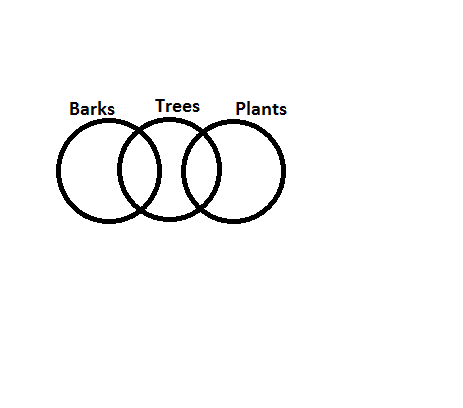According to the diagram, only option A is true.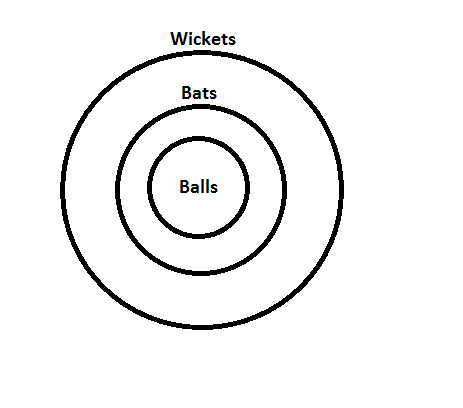Conclusion 1 cannot be deduced from the given information.
The same mid-fielder could have score more than 1 goal. So, the composition of the team does not depend on the number of goals scored by mid-fielders => Conclusion II is wrong.
=> Option C is the answer.

The given situation is highlighted as shown. Only conclusion 1 follows.There are two possibilities in this scenario. Either of them can follow.If all apples are oranges and no mango is an orange, it means no apple is an orange.

Only 2 possibilities follow as shown.Hence we can say for sure that some fruits are flowers.

The conclusion would be true if all android phones are made by Samsung. Hence, option D.

According to the figure, neither of the conclusions follows.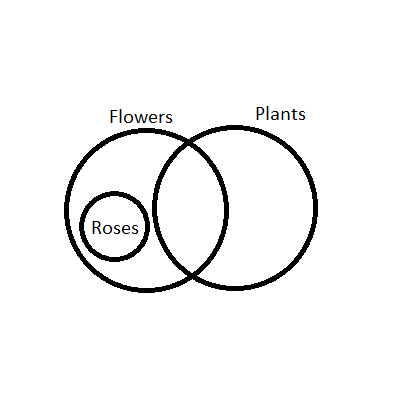According to the figure, only conclusion I follows.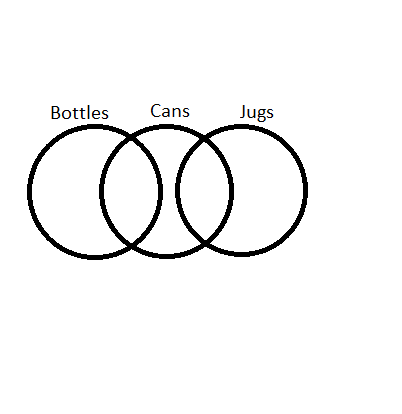The Venn Diagram below satisfies all the three statements and hence explains the syllogism. We can observe that at least some convicts are politicians but it is not necessary that at least some of the honest are criminals.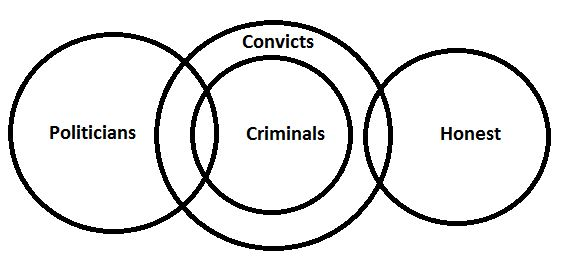So, only conclusion (1) is implied.

The Venn diagram that represents all the statements is shown below:
Since “All officers are tailors.”, we can conclude that at least some tailors are officers. So, conclusion 3 is implied. We can also conclude from the Venn Diagram that at least some clerks as well as managers are tailors.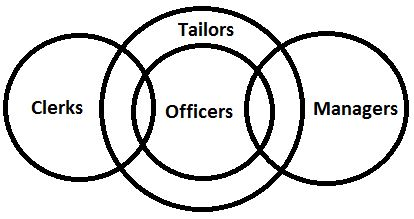So, all the three conclusions are implied.